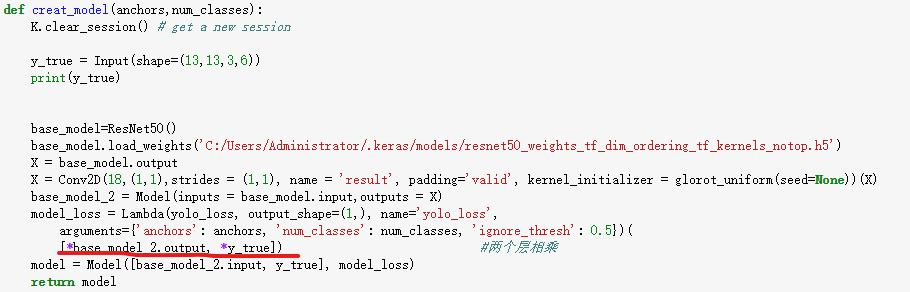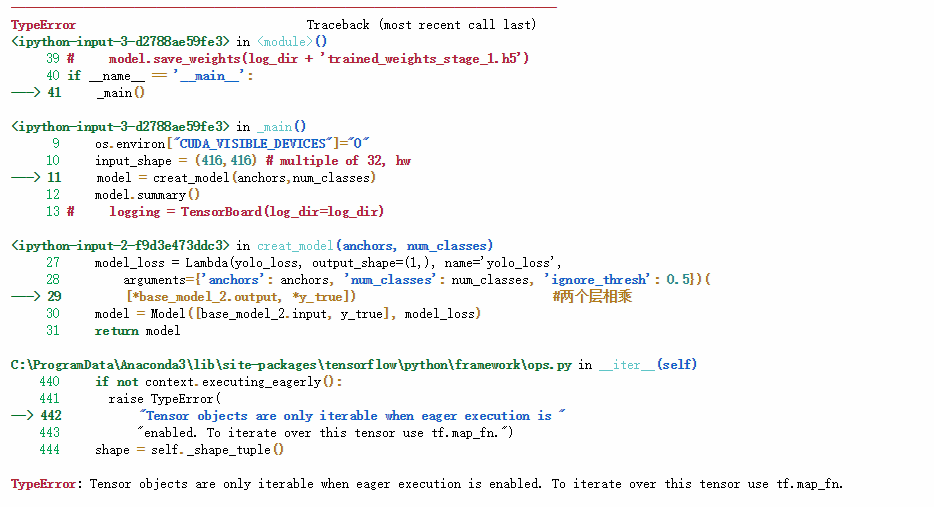Keras使用Lambda搭建最后一层的时候出错，望指教？

50，使用Lambda层来构建最后的loss层，loss公式是借鉴的yolo v3的源代码稍加修改，keras进行自定义层时如何使用一个核与上一层的所有feature map做运算？？

rt 比如说，我想自定义一个层，用一个核来跟上一层的特征图中的32个二维矩阵分别卷积，要怎么实现？假如有代码示例就最好啦 假如说输入是（batch_size,height,width,channel）,这边的batch_size这一维要怎么处理？

keras中如何对网络的某一层参数进行修改？

keras如何搭建跨层神经网络

keras 提示出错 初学者 不明白为什么

from keras.layers import Input, Dense, merge from keras.models import Model from keras import backend as K a = Input(shape=(2,), name='a') b = Input(shape=(2,), name='b') a_rotated = Dense(2, activation='linear')(a) def cosine(x): axis = len(x._keras_shape)-1 dot = lambda a, b: K.batch_dot(a, b, axes=axis) return dot(x, x) / K.sqrt(dot(x, x) * dot(x, x)) cosine_sim = merge([a_rotated, b], mode=cosine, output_shape=lambda x: x[:-1]) model = Model(input=[a, b], output=[cosine_sim]) model.compile(optimizer='sgd', loss='mse') import numpy as np a_data = np.asarray([[0, 1], [1, 0], [0, -1], [-1, 0]]) b_data = np.asarray([[1, 0], [0, -1], [-1, 0], [0, 1]]) targets = np.asarray([1, 1, 1, 1]) model.fit([a_data, b_data], [targets], nb_epoch=1000) print(model.layers.W.get_value()) 这段代码有问题

![图片说明](https://img-ask.csdn.net/upload/201906/19/1560958477_965287.jpg) 我想从一个预训练的卷积神经网络的不同层中提取特征，然后把这些不同层的特征拼接在一起，实现如上图一样的网络结构，我写的代码如下 ``` base_model = VGGFace(model='resnet50', include_top=False) model1 = base_model model2 = base_model input1 = Input(shape=(197,197,3)) model1_out = model1.layers[-12].output model1_in = model1.layers.output model1 = Model(model1_in,model1_out) x1 = model1(input1) x1 = GlobalMaxPool2D()(x1) x2 = model2(input1) x2 = GlobalMaxPool2D()(x2) out = Concatenate(axis=-1)([x1,x2]) out = Dense(1,activation='sigmoid')(out) model3 = Model([input1,input2],out) from keras.utils import plot_model plot_model(model3,"model3.png") import matplotlib.pyplot as plt img = plt.imread('model3.png') plt.imshow(img) ``` 但模型可视化显示如下，两个网络的权值并不共享。![图片说明](https://img-ask.csdn.net/upload/201906/19/1560959263_500375.png)

tensorflow和keras一设置激活函数好像就是会默认设置一整层所有节点都会是同一个激活函数，请问要如何实现同一层不同节点有不同激活函数？

keras用2层cnn和2层全连接搭建食物图像识别的神经网络acc一直在50上不去。

keras如何为已经训练好的模型添加层？

keras搭的模型，训练时候，使用相同的loss和metrics，但是输出却不一样，为什么会出现这种情况呀

Keras实现LSTM进行情感分析的问题，如何正确增加隐层

keras多GPU训练，其中一块无法调用

Keras里，怎么在构建的Model类模型后面加层？

Keras如何在自定义loss函数的时候乘或者加上一个变化的值？

keras使用报出OMP问题

```python batch_size = 128 original_dim = 100 #25*4 latent_dim = 16 # z的维度 intermediate_dim = 256 # 中间层的维度 nb_epoch = 50 # 训练轮数 epsilon_std = 1.0 # 重参数 #my tips:encoding x = Input(batch_shape=(batch_size,original_dim)) h = Dense(intermediate_dim, activation='relu')(x) z_mean = Dense(latent_dim)(h) # mu z_log_var = Dense(latent_dim)(h) # sigma #my tips:Gauss sampling,sample Z def sampling(args): # 重采样 z_mean, z_log_var = args epsilon = K.random_normal(shape=(128, 16), mean=0., stddev=1.0) return z_mean + K.exp(z_log_var / 2) * epsilon # note that "output_shape" isn't necessary with the TensorFlow backend # my tips:get sample z(encoded) z = Lambda(sampling, output_shape=(latent_dim,))([z_mean, z_log_var]) # we instantiate these layers separately so as to reuse them later decoder_h = Dense(intermediate_dim, activation='relu') # 中间层 decoder_mean = Dense(original_dim, activation='sigmoid') # 输出层 h_decoded = decoder_h(z) x_decoded_mean = decoder_mean(h_decoded) #my tips:loss(restruct X)+KL def vae_loss(x, x_decoded_mean): xent_loss = original_dim * objectives.binary_crossentropy(x, x_decoded_mean) kl_loss = - 0.5 * K.mean(1 + z_log_var - K.square(z_mean) - K.exp(z_log_var), axis=-1) return xent_loss + kl_loss vae = Model(x, x_decoded_mean) vae.compile(optimizer='rmsprop', loss=vae_loss) vae.fit(x_train, x_train, shuffle=True, epochs=nb_epoch, verbose=2, batch_size=batch_size, validation_data=(x_valid, x_valid)) vae.save(path+'//VAE.h5') ``` 一段搭建VAE结构的代码，在保存模型后调用时先是出现了sampling中一些全局变量未定义的问题，将变量改为确定数字后又出现了vae_loss函数未定义的问题（unknown loss function: vae_loss) 个人认为是模型中自定义的函数在保存上出现问题，但是也不知道怎么解决。刚刚上手keras和tensorflow这些框架，很多问题是第一次遇到，麻烦大神们帮帮忙！感谢！

linux下利用/proc进行进程树的打印

Python数据分析与挖掘

92讲视频课+16大项目实战+源码+￥800元课程礼包+讲师社群1V1答疑+社群闭门分享会=99元 &nbsp; 为什么学习数据分析？ &nbsp; &nbsp; &nbsp; 人工智能、大数据时代有什么技能是可以运用在各种行业的？数据分析就是。 &nbsp; &nbsp; &nbsp; 从海量数据中获得别人看不见的信息，创业者可以通过数据分析来优化产品，营销人员可以通过数据分析改进营销策略，产品经理可以通过数据分析洞察用户习惯，金融从业者可以通过数据分析规避投资风险，程序员可以通过数据分析进一步挖掘出数据价值，它和编程一样，本质上也是一个工具，通过数据来对现实事物进行分析和识别的能力。不管你从事什么行业，掌握了数据分析能力，往往在其岗位上更有竞争力。 &nbsp;&nbsp; 本课程共包含五大模块： 一、先导篇： 通过分析数据分析师的一天，让学员了解全面了解成为一个数据分析师的所有必修功法，对数据分析师不在迷惑。 &nbsp; 二、基础篇： 围绕Python基础语法介绍、数据预处理、数据可视化以及数据分析与挖掘......这些核心技能模块展开，帮助你快速而全面的掌握和了解成为一个数据分析师的所有必修功法。 &nbsp; 三、数据采集篇： 通过网络爬虫实战解决数据分析的必经之路：数据从何来的问题，讲解常见的爬虫套路并利用三大实战帮助学员扎实数据采集能力，避免没有数据可分析的尴尬。 &nbsp; 四、分析工具篇： 讲解数据分析避不开的科学计算库Numpy、数据分析工具Pandas及常见可视化工具Matplotlib。 &nbsp; 五、算法篇： 算法是数据分析的精华，课程精选10大算法，包括分类、聚类、预测3大类型，每个算法都从原理和案例两个角度学习，让你不仅能用起来，了解原理，还能知道为什么这么做。

Only老K说-爬取妹子图片（简单入门）

linux“开发工具三剑客”速成攻略

Python代码实现飞机大战

Python数据清洗实战入门

2019 Python开发者日-培训

apache-jmeter-5.1.1(Requires Java 8+).zip

。Apache JMeter 5.1.1 (Requires Java 8+)，需要jdk8以上的版本。

Python可以这样学（第四季：数据分析与科学计算可视化）

Java基础知识面试题（2020最新版）

2019 AI开发者大会

2019 AI开发者大会(AI ProCon 2019)是由中国IT社区CSDN主办的AI技术与产业年度盛会。多年经验淬炼，如今蓄势待发：2019年9月6-7日，大会将有近百位中美顶尖AI专家、知名企业代表以及千余名AI开发者齐聚北京，进行技术解读和产业论证。我们不空谈口号，只谈技术，诚挚邀请AI业内人士一起共铸人工智能新篇章！

Python数据挖掘简易入门

&nbsp; &nbsp; &nbsp; &nbsp; 本课程为Python数据挖掘方向的入门课程，课程主要以真实数据为基础，详细介绍数据挖掘入门的流程和使用Python实现pandas与numpy在数据挖掘方向的运用，并深入学习如何运用scikit-learn调用常用的数据挖掘算法解决数据挖掘问题，为进一步深入学习数据挖掘打下扎实的基础。

C/C++学习指南全套教程

C/C++学习的全套教程，从基本语法，基本原理，到界面开发、网络开发、Linux开发、安全算法，应用尽用。由毕业于清华大学的业内人士执课，为C/C++编程爱好者的教程。

2021考研数学张宇基础30讲.pdf

<p> 限时福利限时福利，<span>15000+程序员的选择！</span> </p> <p> 购课后添加学习助手（微信号：csdn590），按提示消息领取编程大礼包！并获取讲师答疑服务！ </p> <p> <br> </p> <p> 套餐中一共包含5门程序员必学的数学课程（共47讲） </p> <p> 课程1：《零基础入门微积分》 </p> <p> 课程2：《数理统计与概率论》 </p> <p> 课程3：《代码学习线性代数》 </p> <p> 课程4：《数据处理的最优化》 </p> <p> 课程5：《马尔可夫随机过程》 </p> <p> <br> </p> <p> 哪些人适合学习这门课程？ </p> <p> 1）大学生，平时只学习了数学理论，并未接触如何应用数学解决编程问题； </p> <p> 2）对算法、数据结构掌握程度薄弱的人，数学可以让你更好的理解算法、数据结构原理及应用； </p> <p> 3）看不懂大牛代码设计思想的人，因为所有的程序设计底层逻辑都是数学； </p> <p> 4）想学习新技术，如：人工智能、机器学习、深度学习等，这门课程是你的必修课程； </p> <p> 5）想修炼更好的编程内功，在遇到问题时可以灵活的应用数学思维解决问题。 </p> <p> <br> </p> <p> 在这门「专为程序员设计的数学课」系列课中，我们保证你能收获到这些:<br> <br> <span> </span> </p> <p class="ql-long-24357476"> <span class="ql-author-24357476">①价值300元编程课程大礼包</span> </p> <p class="ql-long-24357476"> <span class="ql-author-24357476">②应用数学优化代码的实操方法</span> </p> <p class="ql-long-24357476"> <span class="ql-author-24357476">③数学理论在编程实战中的应用</span> </p> <p class="ql-long-24357476"> <span class="ql-author-24357476">④程序员必学的5大数学知识</span> </p> <p class="ql-long-24357476"> <span class="ql-author-24357476">⑤人工智能领域必修数学课</span> </p> <p> <br> 备注：此课程只讲程序员所需要的数学，即使你数学基础薄弱，也能听懂，只需要初中的数学知识就足矣。<br> <br> 如何听课？ </p> <p> 1、登录CSDN学院 APP 在我的课程中进行学习； </p> <p> 2、登录CSDN学院官网。 </p> <p> <br> </p> <p> 购课后如何领取免费赠送的编程大礼包和加入答疑群？ </p> <p> 购课后，添加助教微信：<span> csdn590</span>，按提示领取编程大礼包，或观看付费视频的第一节内容扫码进群答疑交流！ </p> <p> <img src="https://img-bss.csdn.net/201912251155398753.jpg" alt=""> </p>

DDR5_Draft_Spec_Rev05c.pdf

DDR5 spec

Java面试史上最全的JAVA专业术语面试100问 (前1-50)

C/C++跨平台研发从基础到高阶实战系列套餐

Python界面版学生管理系统

2019数学建模A题高压油管的压力控制 省一论文即代码

2019数学建模A题高压油管的压力控制省一完整论文即详细C++和Matlab代码，希望对同学们有所帮助

4小时玩转微信小程序——基础入门与微信支付实战